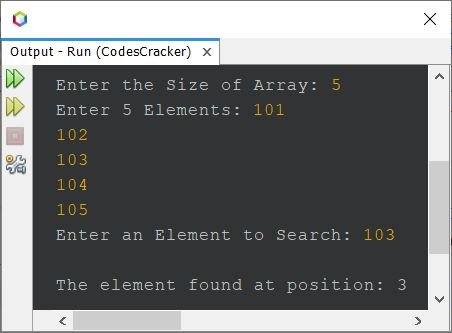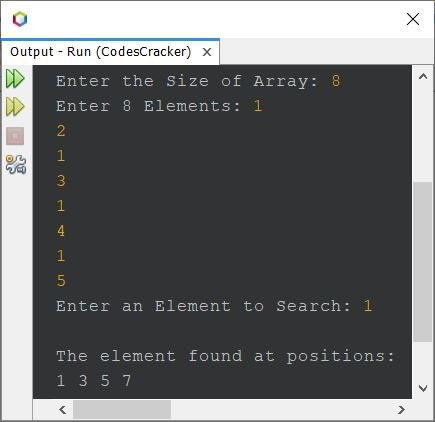# Java Program for Linear Search

This article covers multiple programs in Java that find and prints the position(s) of an element in an array entered by user at run-time of the program, using linear search technique. List of programs covered in this article:

• Simple linear search program based on 10 elements
• Linear search program based on n elements
• Linear search with duplicate elements

If you're not aware about, how the linear search works ?
then refer to Linear Search Algorithm and Example. Now let's create the program.

## Linear Search in Java

The question is, write a Java program to perform linear search. The program given below is its answer:

```import java.util.Scanner;

public class CodesCracker
{
public static void main(String[] args)
{
int i, num, pos=0;
int[] arr = new int;
Scanner s = new Scanner(System.in);

System.out.print("Enter 10 Elements: ");
for(i=0; i<10; i++)
arr[i] = s.nextInt();

System.out.print("Enter an Element to Search: ");
num = s.nextInt();

for(i=0; i<10; i++)
{
if(num==arr[i])
{
pos = i+1;
break;
}
}
if(pos==0)
else
System.out.println("\nThe element found at position: " +pos);
}
}```

The snapshot given below shows the sample run of above program, with user input 1, 3, 4, 6, 7, 8, 10, 12, 15, 18 as 10 elements and 10 as element to search:### Linear Search in Java based on n Elements

This is the same program as of previous one. But this program allows user to define the size of array too, along with its elements

```import java.util.Scanner;

public class CodesCracker
{
public static void main(String[] args)
{
int i, pos=0;
Scanner s = new Scanner(System.in);

System.out.print("Enter the Size of Array: ");
int n = s.nextInt();
int[] arr = new int[n];
System.out.print("Enter " +n+" Elements: ");
for(i=0; i<n; i++)
arr[i] = s.nextInt();

System.out.print("Enter an Element to Search: ");
int num = s.nextInt();

for(i=0; i<n; i++)
{
if(num==arr[i])
{
pos = i+1;
break;
}
}
if(pos==0)
else
System.out.println("\nThe element found at position: " +pos);
}
}```

The sample run with user input 5 as size, 101, 102, 103, 104, 105 as five elements, and 103 as element to search, is shown in the snapshot given below:## Linear Search with Duplicate Elements in Java

While creating the program given above, I thought, what if user enters an element that are available multiple times in the given array. Therefore, in that case the program given above will not produce the perfect output.

So I decided to create another program, that of course uses linear search to find and print all the positions of an element, in an array. This program also works for unique element.

```import java.util.Scanner;

public class CodesCracker
{
public static void main(String[] args)
{
int i, posIndex=0;
Scanner s = new Scanner(System.in);

System.out.print("Enter the Size of Array: ");
int n = s.nextInt();
int[] arr = new int[n];
int[] pos = new int[n];
System.out.print("Enter " +n+" Elements: ");
for(i=0; i<n; i++)
arr[i] = s.nextInt();

System.out.print("Enter an Element to Search: ");
int num = s.nextInt();

for(i=0; i<n; i++)
{
if(num==arr[i])
{
pos[posIndex] = i+1;
posIndex++;
}
}
if(posIndex==0)
else if(posIndex==1)
System.out.println("\nThe element found at position: " +pos);
else
{
System.out.println("\nThe element found at positions: ");
for(i=0; i<posIndex; i++)
System.out.print(pos[i]+ " ");
}
}
}```

Here is its sample run with user input 8 as size, 1, 2, 1, 3, 1, 4, 1, 5 as eight elements, and 1 as element to search, find and print the positions where the element is available in the array:#### Same Program in Other Languages

Java Online Test

« Previous Program Next Program »